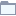# Bayes

This category contains 6 nodes.

##### Naive Bayes Learner

Creates a naive Bayes model from the given classified data.

##### Naive Bayes LearnerDeprecated

Creates a naive Bayes model from the given classified data.

##### Naive Bayes LearnerDeprecated

Creates a naive Bayes model from the given classified data.

##### Naive Bayes PredictorStreamable

Uses the PMML naive Bayes model from the naive Bayes learner to predict the class membership of each row in the input data.

##### Naive Bayes PredictorDeprecated

Uses the naive Bayes model from the naive Bayes learner to predict the class membership of each row in the input data.

##### Naive Bayes PredictorStreamableDeprecated

Uses the PMML naive Bayes model from the naive Bayes learner to predict the class membership of each row in the input data.